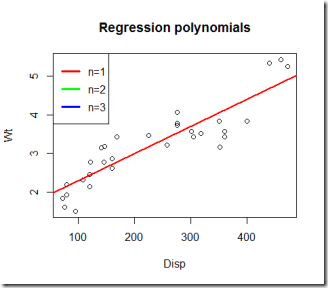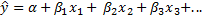Want to share your content on R-bloggers? click here if you have a blog, or here if you don't.

Linear regression models are one the simplest and yet a very powerful models you can use in R to fit observed data and try to predict quantitative phenomena.

Say you know that a certain variable y is somewhat correlated with a certain variable x and you can reasonably get an idea of what y would be given x. A class example is the price of houses (y) and square meters (x). It is reasonable to assume that, within a certain range and given the same location, square meters are correlated with the price of the house. As a first rough approximation one could try out the hypothesis that price is directly proportional to square meters.

Now what if you would like to predict the possible price of a flat of 80 square meters? Well, an initial approach could be gathering the data of houses in the same location and with similar characteristics and then fit a model.

A linear model with one predicting variable might be the following:

Alpha and Beta can be found by looking for for the two values that minimise the error of the model relative to the observed data. Basically the procedure of finding the two coefficient is equivalent to finding the “closest” line to our data. Of course this will still be an estimate and it will probably not match any of the observed values.

In R fitting these models is quite easy, let’s code an example using the mtcars dataset and setting x = displacement, y = weight. It looks reasonable to assume that displacement and weight be correlated.So far our assumption seems reasonable.

Let’s fit the model.

Overall the fitted line (in red) seems to be a good fit to our data and this is confirmed by the R squared value which is > 0.7. There is no minimum value for the R squared coefficient to be significant, however a value less than 0.3 usually indicates a bad fit and you should probably change the model you are using (R squared varies between 0 and 1). Take a close look at the p values too: a low value of the p value signals that the variable (in this case Disp) is statistically significant. Indeed the confidence intervals for the estimated coefficient of mpg do not include 0.Sometimes the relation between two variables is all but linear, therefore a better approach could be the use of quadratic or cubic model as is used below.

The quadratic model seems pretty useless in this case since it is not more helpful than the original red line. The cubic model seems like a better fit to our data compared to the two previous models and perhaps could be the one that we were looking for.

In principle you could go on and fit higher polynomials to your data, however this might not be the optimal choice because you have to remember that you are looking at a fraction of the whole data available and perhaps some of it was distorted by measuring errors or other kind of errors and by trying to fit your model as close as you can to the data, you are implicitly setting it to be very sensitive to these errors (you are kind of overfitting it). Depending on what you are modelling you have to choose between this trade-off of good fit and a more sensitive model.Now what if you have more information available? Well it is usually good to use it and put it into your model: R squared will surely increase however we will need to check the p values for statistical significance and the F stastistics.

If, for instance, you could use other variables such as Gross horsepower (hp), Rear axle ratio (drat) and  Miles/(US) gallon (mpg) you could fit a linear model that takes into account all these new variablesOf course now the graph is much more difficult to visualise, however we can plot y hat against x1 for instance.

Furthermore, by taking a look at the summary of the model, we can understand which variables influence the most the outcome of the model and which don’t. This last model is probably better than the cubic model we fitted above because it uses more information. In R is quite easy and fun to play around with these models and combine them together.